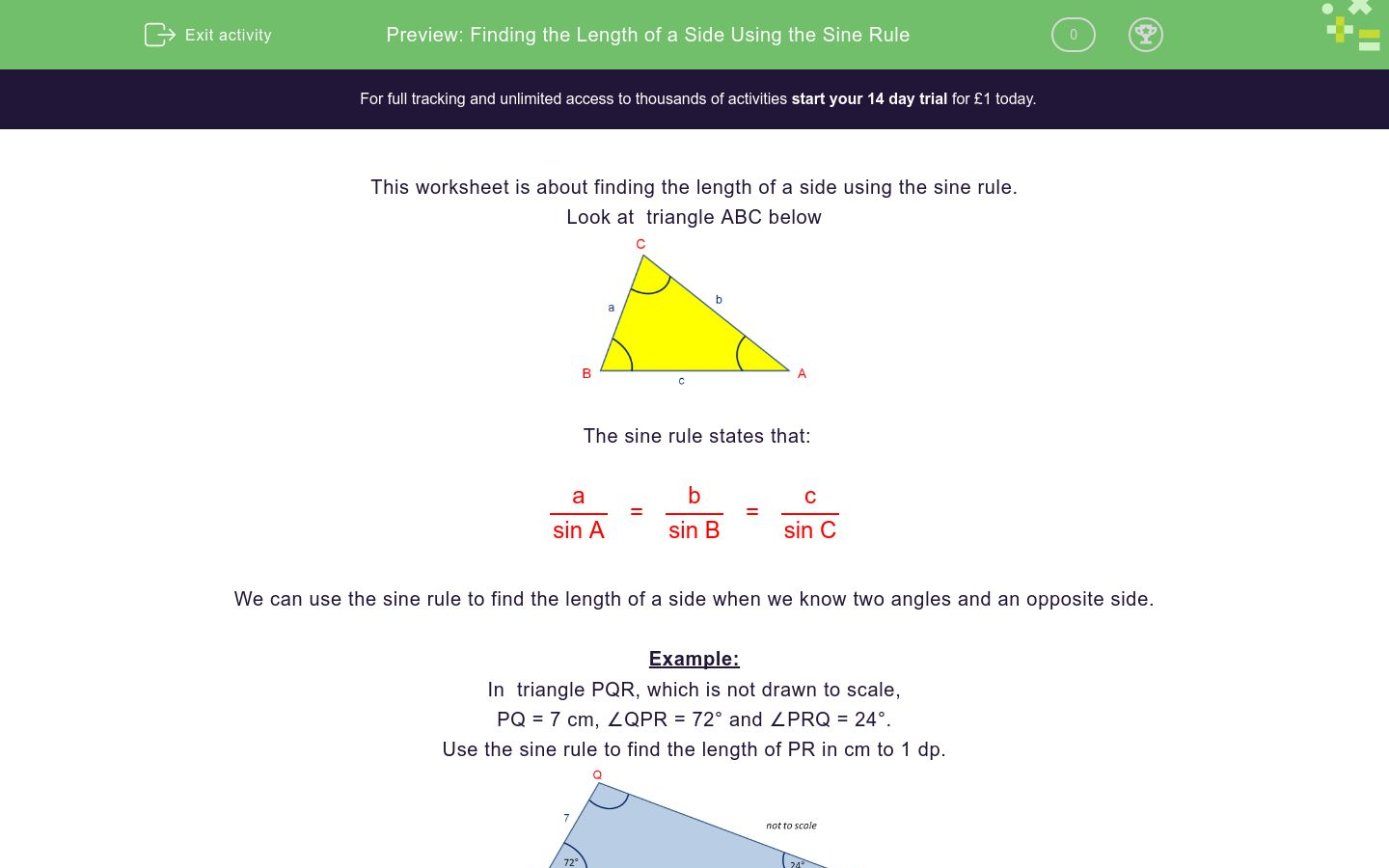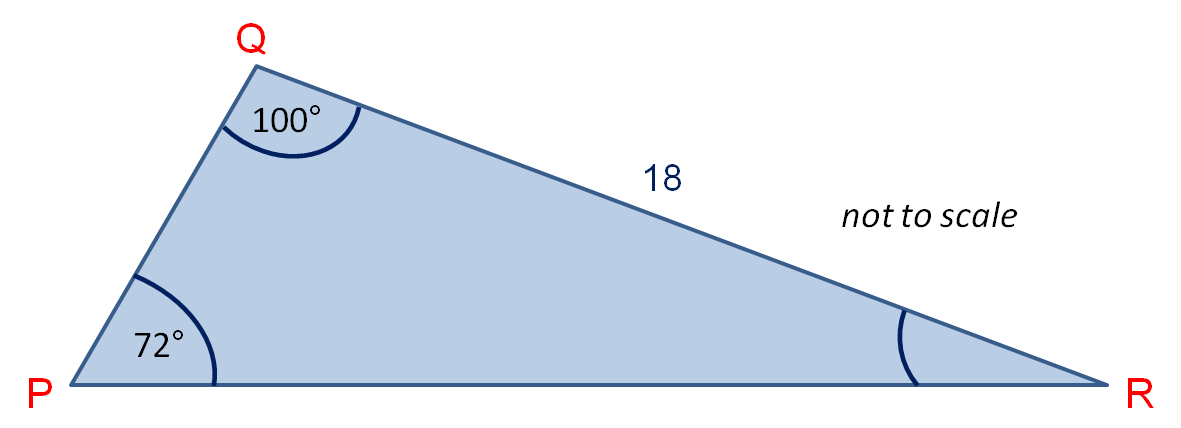# Finding the Length of a Side Using the Sine Rule

In this worksheet, students find the length of a side given two angles and an opposite side in a triangle by using the sine rule.Key stage:  KS 4

Curriculum topic:  Geometry and Measures

Curriculum subtopic:  Know the Exact Values of Sin, Cos and Tan

Difficulty level:### QUESTION 1 of 10

This worksheet is about finding the length of a side using the sine rule.

Look at  triangle ABC belowThe sine rule states that:

 a = b = c sin A sin B sin C

We can use the sine rule to find the length of a side when we know two angles and an opposite side.

Example:

In  triangle PQR, which is not drawn to scale,

PQ = 7 cm, ∠QPR = 72° and ∠PRQ = 24°.

Use the sine rule to find the length of PR in cm to 1 dp.First we must find ∠PQR = 180° - 72° - 24° = 84°.

Using the sine rule,

 PR = 7 sin 84° sin 24°

 PR = 7 x sin 84° sin 24°

PR = 7 x sin 84° ÷ sin 24°

PR = 17.1 cm

In  triangle PQR, which is not drawn to scale,

PQ = 7 cm, ∠QPR = 72° and ∠PRQ = 24°

Use the sine rule to find the length of QR in cm to 1 dp.

(Just write the number)In  triangle PQR, which is not drawn to scale,

QR = 18 cm, ∠QPR = 72° and ∠PQR = 100°

Use the sine rule to find the length of PR in cm to 1 dp.

(Just write the number)In  triangle PQR, which is not drawn to scale,

PQ = 9 cm, ∠QPR = 32° and ∠PRQ = 38°

Use the sine rule to find the length of QR in cm to 1 dp.

(Just write the number)In  triangle PQR, which is not drawn to scale,

PR = 19 cm, ∠PQR = 102° and ∠PRQ = 37°

Use the sine rule to find the length of PQ in cm to 1 dp.

(Just write the number)In  triangle PQR, which is not drawn to scale,

QR = 18 cm, ∠QPR = 72° and ∠PQR = 100°

Use the sine rule to find the length of PQ in cm to 1 dp.

(Just write the number)In  triangle PQR, which is not drawn to scale,

PQ = 9 cm, ∠QPR = 32° and ∠PRQ = 38°

Use the sine rule to find the length of PR in cm to 1 dp.

(Just write the number)In  triangle PQR, which is not drawn to scale,

PR = 19 cm, ∠PQR = 102° and ∠PRQ = 37°

Use the sine rule to find the length of QR in cm to 1 dp.

(Just write the number)In  triangle PQR, which is not drawn to scale,

PR = 12 cm, ∠PQR = 95° and ∠QPR = 62°

Use the sine rule to find the length of QR in cm to 1 dp.

(Just write the number)In  triangle PQR, which is not drawn to scale,

PR = 12 cm, ∠PQR = 95° and ∠QPR = 62°

Use the sine rule to find the length of PQ in cm to 1 dp.

(Just write the number)In  triangle ABC, which is not drawn to scale,

b = 6.5 cm, ∠CBA = 65° and ∠BCA = 65°

Use the sine rule to find the length of AB in cm to 1 dp.

(Just write the number)• Question 1

In  triangle PQR, which is not drawn to scale,

PQ = 7 cm, ∠QPR = 72° and ∠PRQ = 24°

Use the sine rule to find the length of QR in cm to 1 dp.

(Just write the number)16.4
EDDIE SAYS
 QR = 7 sin 72° sin 24°
• Question 2

In  triangle PQR, which is not drawn to scale,

QR = 18 cm, ∠QPR = 72° and ∠PQR = 100°

Use the sine rule to find the length of PR in cm to 1 dp.

(Just write the number)18.6
EDDIE SAYS
 PR = 18 sin 100° sin 72°
• Question 3

In  triangle PQR, which is not drawn to scale,

PQ = 9 cm, ∠QPR = 32° and ∠PRQ = 38°

Use the sine rule to find the length of QR in cm to 1 dp.

(Just write the number)7.7
EDDIE SAYS
 QR = 9 sin 32° sin 38°
• Question 4

In  triangle PQR, which is not drawn to scale,

PR = 19 cm, ∠PQR = 102° and ∠PRQ = 37°

Use the sine rule to find the length of PQ in cm to 1 dp.

(Just write the number)11.7
EDDIE SAYS
 PQ = 19 sin 37° sin 102°
• Question 5

In  triangle PQR, which is not drawn to scale,

QR = 18 cm, ∠QPR = 72° and ∠PQR = 100°

Use the sine rule to find the length of PQ in cm to 1 dp.

(Just write the number)2.6
EDDIE SAYS
Missing angle = 180° - 100° - 72° = 8°
 PQ = 18 sin 8° sin 72°
• Question 6

In  triangle PQR, which is not drawn to scale,

PQ = 9 cm, ∠QPR = 32° and ∠PRQ = 38°

Use the sine rule to find the length of PR in cm to 1 dp.

(Just write the number)13.7
EDDIE SAYS
Missing angle = 180° - 32° - 38° = 110°
 PR = 9 sin 110° sin 38°
• Question 7

In  triangle PQR, which is not drawn to scale,

PR = 19 cm, ∠PQR = 102° and ∠PRQ = 37°

Use the sine rule to find the length of QR in cm to 1 dp.

(Just write the number)12.7
EDDIE SAYS
Missing angle = 180° - 102° - 37° = 41°
 QR = 19 sin 41° sin 102°
• Question 8

In  triangle PQR, which is not drawn to scale,

PR = 12 cm, ∠PQR = 95° and ∠QPR = 62°

Use the sine rule to find the length of QR in cm to 1 dp.

(Just write the number)10.6
EDDIE SAYS
 QR = 12 sin 62° sin 95°
• Question 9

In  triangle PQR, which is not drawn to scale,

PR = 12 cm, ∠PQR = 95° and ∠QPR = 62°

Use the sine rule to find the length of PQ in cm to 1 dp.

(Just write the number)4.7
EDDIE SAYS
Missing angle = 180° - 95° - 62° = 23°
 PQ = 12 sin 23° sin 95°
• Question 10

In  triangle ABC, which is not drawn to scale,

b = 6.5 cm, ∠CBA = 65° and ∠BCA = 65°

Use the sine rule to find the length of AB in cm to 1 dp.

(Just write the number)6.5
EDDIE SAYS
 c = 6.5 sin 65° sin 65°

The triangle is isosceles
---- OR ----

Sign up for a £1 trial so you can track and measure your child's progress on this activity.

### What is EdPlace?

We're your National Curriculum aligned online education content provider helping each child succeed in English, maths and science from year 1 to GCSE. With an EdPlace account you’ll be able to track and measure progress, helping each child achieve their best. We build confidence and attainment by personalising each child’s learning at a level that suits them.

Start your £1 trial